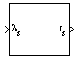Documentation

# Radius at Geocentric Latitude

Estimate radius of ellipsoid planet at geocentric latitude

## Library

Flight Parameters## Description

The Radius at Geocentric Latitude block estimates the radius $\left({r}_{s}\right)$ of an ellipsoid planet at a particular geocentric latitude $\left({\lambda }_{s}\right)$.The following equation estimates the ellipsoid radius $\left({r}_{s}\right)$ using flattening $\left(\overline{f}\right)$, geocentric latitude $\left({\overline{\lambda }}_{s}\right)$, and equatorial radius $\left(\overline{R}\right)$.

`${r}_{s}=\sqrt{\frac{{R}^{2}}{1+\left[1/{\left(1-f\right)}^{2}-1\right]{\mathrm{sin}}^{2}{\lambda }_{s}}}$`

## Parameters

Units

Specifies the parameter and output units:

UnitsEquatorial RadiusRadius at Geocentric Latitude
`Metric (MKS)`MetersMeters
`English`FeetFeet

This option is only available when Planet model is set to `Earth (WGS84)`.

Planet model

Specifies the planet model to use:

`Custom`

`Earth (WGS84)`

Flattening

Specifies the flattening of the planet. This option is only available with Planet model set to `Custom`.

Equatorial radius of planet

Specifies the radius of the planet at its equator. This option is only available with Planet model set to `Custom`.

## Inputs and Outputs

InputDimension TypeDescription

First

Contains the geocentric latitude, in degrees.
OutputDimension TypeDescription

First

Contains the radius of planet at geocentric latitude, in the same as the units as flattening.

## References

Stevens, B. L., and F. L. Lewis, Aircraft Control and Simulation, John Wiley & Sons, New York, 1992.

Zipfel, P. H., Modeling and Simulation of Aerospace Vehicle Dynamics, AIAA Education Series, Reston, Virginia, 2000.

#### Introduced before R2006a

##### SupportGet trial now